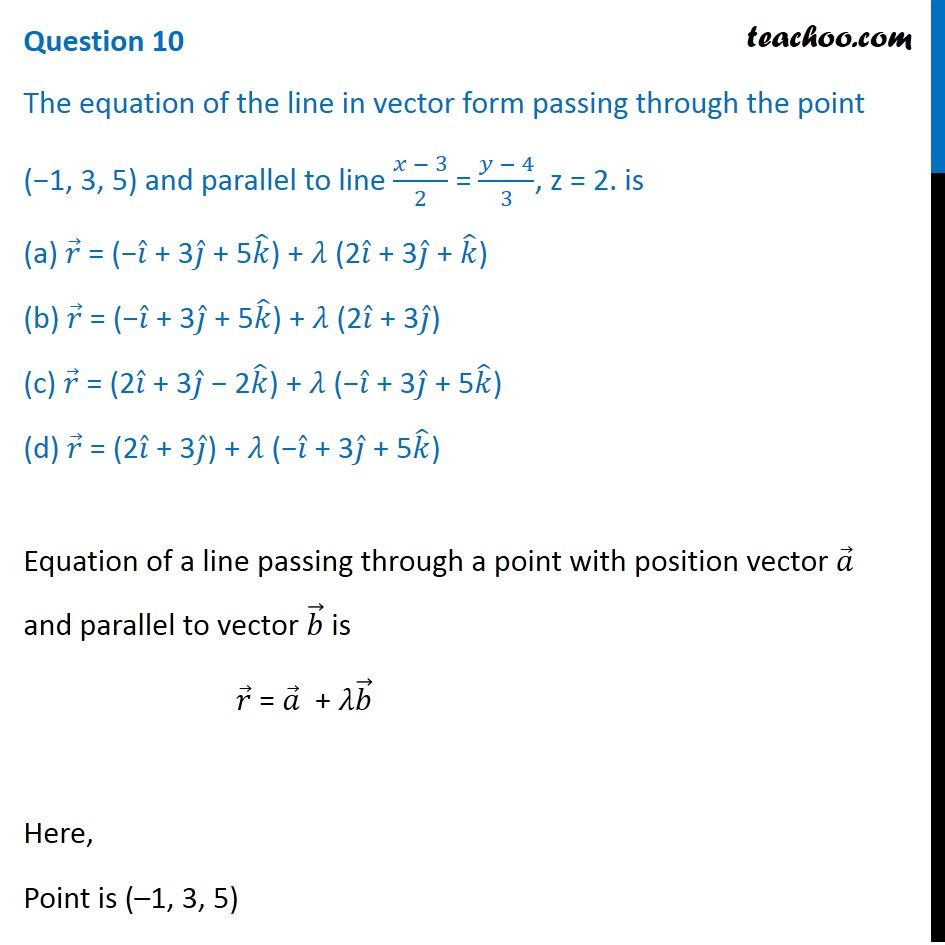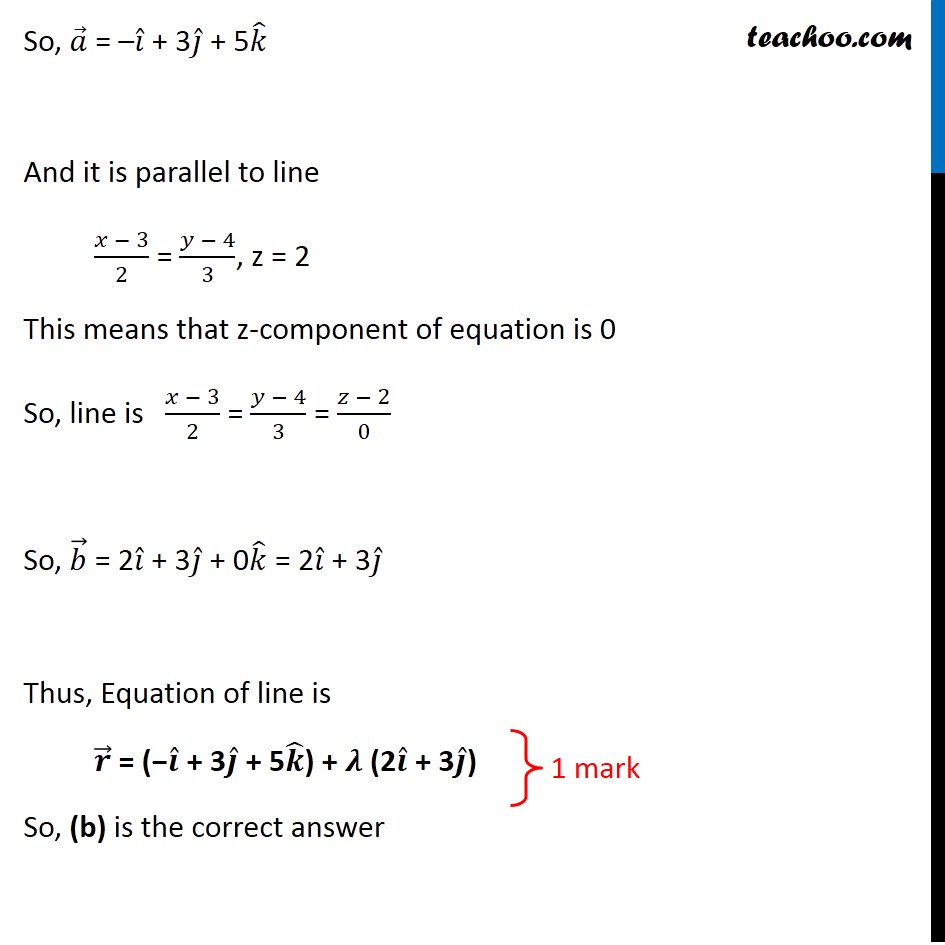CBSE Class 12 Sample Paper for 2020 Boards

Class 12
Solutions of Sample Papers and Past Year Papers - for Class 12 Boards

## (d) r  = (2i  + 3j  ) + λ (−i  + 3j  + 5k  )Learn in your speed, with individual attention - Teachoo Maths 1-on-1 Class

### Transcript

Question 10 The equation of the line in vector form passing through the point (−1, 3, 5) and parallel to line (𝑥 − 3)/2 = (𝑦 − 4)/3, z = 2. is (a) 𝑟 ⃗ = (−𝑖 ̂ + 3𝑗 ̂ + 5𝑘 ̂) + 𝜆 (2𝑖 ̂ + 3𝑗 ̂ + 𝑘 ̂) (b) 𝑟 ⃗ = (−𝑖 ̂ + 3𝑗 ̂ + 5𝑘 ̂) + 𝜆 (2𝑖 ̂ + 3𝑗 ̂) (c) 𝑟 ⃗ = (2𝑖 ̂ + 3𝑗 ̂ − 2𝑘 ̂) + 𝜆 (−𝑖 ̂ + 3𝑗 ̂ + 5𝑘 ̂) (d) 𝑟 ⃗ = (2𝑖 ̂ + 3𝑗 ̂) + 𝜆 (−𝑖 ̂ + 3𝑗 ̂ + 5𝑘 ̂) Equation of a line passing through a point with position vector 𝑎 ⃗ and parallel to vector 𝑏 ⃗ is 𝑟 ⃗ = 𝑎 ⃗ + 𝜆𝑏 ⃗ Here, Point is (–1, 3, 5) So, 𝑎 ⃗ = –𝑖 ̂ + 3𝑗 ̂ + 5𝑘 ̂ And it is parallel to line (𝑥 − 3)/2 = (𝑦 − 4)/3, z = 2 This means that z-component of equation is 0 So, line is (𝑥 − 3)/2 = (𝑦 − 4)/3 = (𝑧 − 2)/0 So, 𝑏 ⃗ = 2𝑖 ̂ + 3𝑗 ̂ + 0𝑘 ̂ = 2𝑖 ̂ + 3𝑗 ̂ Thus, Equation of line is 𝒓 ⃗ = (−𝒊 ̂ + 3𝒋 ̂ + 5𝒌 ̂) + 𝝀 (2𝒊 ̂ + 3𝒋 ̂) So, (b) is the correct answer